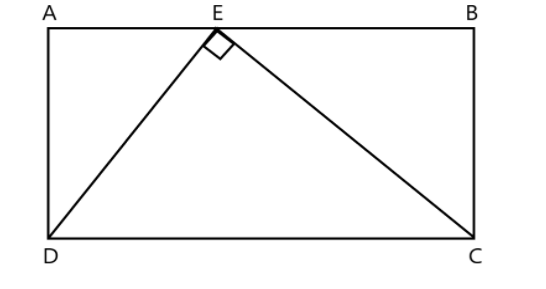Courses
Courses for Kids
Free study material
Free LIVE classes
More# : In the diagram $ABCD$ is a rectangle and point $E$ lies on $AB$. Triangle $DEC$ has $\left| \!{\underline { {DEC} }} \right. = {90^ \circ }$, $DE = 3$ and $EC = 4$. The length of $AD$ isA.2.4B.2.8C.1.8D.3.2

Last updated date: 21st Mar 2023
Total views: 204.9k
Views today: 4.84kVerified
204.9k+ views
Hint: Here in this question, we have to find the length of the side AD in the given rectangular diagram AD, this can be solve by applying a Pythagoras theorem i.e., $hy{p^2} = op{p^2} + ad{j^2}$ in triangles $\Delta DEC$, $\Delta EAD$, and $\Delta EBC$ then further simplify by using a basic arithmetic operation we get the required length of the side AD.

Consider the given diagram $ABCD$ is a rectangular and point E lies on the side AB, and the given line DE and EC which have a length 3 and 4 respectively and the point E makes angle ${90^ \circ }$.
In rectangle $ABCD$, we found three triangles i.e., $\Delta DEC$, $\Delta EAD$ and $\Delta EBC$. E be the common point in all three triangles.
Now, consider the triangle $\Delta DEC$ is a right-angled triangle.
In $\Delta DEC$, $\left| \!{\underline { E }} \right. = {90^ \circ }$
Then by the Pythagoras theorem
$\Rightarrow {\left( {DC} \right)^2} = {\left( {DE} \right)^2} + {\left( {EC} \right)^2}$
Given, $DE = 3$ and $EC = 4$, on substituting we have
$\Rightarrow {\left( {DC} \right)^2} = {\left( 3 \right)^2} + {\left( 4 \right)^2}$
$\Rightarrow {\left( {DC} \right)^2} = 9 + 16$
$\Rightarrow {\left( {DC} \right)^2} = 25$
Taking square root on both side, then
$\Rightarrow DC = \pm \sqrt {25}$
$\Rightarrow DC = \pm 5$
When, measuring the length of any shape we only consider the positive value
$\therefore DC = 5$
Now, consider triangle $\Delta EAD$ is a right-angle triangle
In, $\Delta EAD$, $\left| \!{\underline { A }} \right. = {90^ \circ }$and let take $AE = x$
Then by the Pythagoras theorem
$\Rightarrow {\left( {DE} \right)^2} = {\left( {AD} \right)^2} + {\left( {AE} \right)^2}$
$\Rightarrow {\left( 3 \right)^2} = {\left( {AD} \right)^2} + {\left( x \right)^2}$
$\Rightarrow 9 = {\left( {AD} \right)^2} + {x^2}$
On rearranging, we have
$\Rightarrow {\left( {AD} \right)^2} = 9 - {x^2}$-------(1)
Now, consider triangle $\Delta EBC$ is a right-angle triangle
In, $\Delta EBC$, $\left| \!{\underline { B }} \right. = {90^ \circ }$ and
Let, $EB = AB - AE$
But $AB = DC = 5$, then
$\Rightarrow EB = 5 - x$
Then by the Pythagoras theorem
$\Rightarrow {\left( {EC} \right)^2} = {\left( {BC} \right)^2} + {\left( {EB} \right)^2}$
But $BC = AD$, then
$\Rightarrow {\left( 4 \right)^2} = {\left( {AD} \right)^2} + {\left( {5 - x} \right)^2}$
$\Rightarrow 16 = {\left( {AD} \right)^2} + {\left( {5 - x} \right)^2}$
On applying a algebraic identity: ${\left( {a - b} \right)^2} = {a^2} + {b^2} - 2ab$, then
$\Rightarrow 16 = {\left( {AD} \right)^2} + {5^2} + {x^2} - 10x$
$\Rightarrow 16 = {\left( {AD} \right)^2} + 25 + {x^2} - 10x$
On rearranging, we have
$\Rightarrow {\left( {AD} \right)^2} = 16 - 25 - {x^2} + 10x$
$\Rightarrow {\left( {AD} \right)^2} = 10x - 9 - {x^2}$ ------(2)
From equation (1) and (2), we have
$\Rightarrow 9 - {x^2} = 10x - 9 - {x^2}$
Take variable $x$ terms in to the LHS, then
$\Rightarrow {x^2} - {x^2} - 10x = - 9 - 9$
On simplification, we have
$\Rightarrow - 10x = - 18$
Divide both side by -10, then we get
$\Rightarrow x = \dfrac{{ - 18}}{{ - 10}}$
$\Rightarrow x = 1.8$
Substitute $x$ value in equation (1), then
$\Rightarrow {\left( {AD} \right)^2} = 9 - {\left( {1.8} \right)^2}$
$\Rightarrow {\left( {AD} \right)^2} = 9 - 3.24$
$\Rightarrow {\left( {AD} \right)^2} = 5.76$
Take square root on both side, we have
$\Rightarrow AD = \pm \sqrt {5.76}$
$\Rightarrow AD = \pm 2.4$
$\therefore AD = 2.4$
Hence, the length of the side $AD = 2.4$.
Therefore, Option (A) is correct.
So, the correct answer is “Option A”.

Note: To solve rectangular based problems, remember the properties of triangle i.e., the parallel sides are equal and when triangle is right angle it‘s obey the Pythagoras theorem which stated as “In a right-angled triangle, the square of the hypotenuse side is equal to the sum of squares of the other two sides” i.e., $hy{p^2} = op{p^2} + ad{j^2}$, and main thing is measurement of length of any shape should be in positive.